# RBSE Maths Class 8 Chapter 16: Important Questions and Solutions

RBSE Maths Chapter 16 – Data Handling Class 8 Important questions and solutions can be accessed here. The important questions and solutions of Chapter 16, available at BYJU’S, contain detailed step by step explanations. All these important questions are based on the new pattern prescribed by the RBSE. Students can also get the syllabus and textbooks on RBSE Class 8 solutions.

Chapter 16 of the RBSE Class 8 Maths will help the students to solve problems related to the histogram, frequency distribution table using tally marks, bar graph, pie chart, problems on diagrammatic representation of data, probability of events.

### RBSE Maths Chapter 16: Exercise 16.1 Textbook Important Questions and Solutions

Question 1: Which of the following types of data will need a histogram?

(i) Quantity of different types of cereals available at home.

(ii) Height of all students at your school.

(iii) The number of cars manufactured by 5 companies.

(iv) The number of vehicles passing a busy crossroad between 8:00 A.M. and 2:00 PM.

(v) Distance between home to the school of all students of your class. (in metres)

Solution:

A histogram is used to show the data of (ii), (iv) and (v).

Question 2: Rakesh distinguishes his clothes on the basis of colours and indicates like this – White (W), Red (R), Black (B), Yellow (Y) and Others (X). The list formed is as follows R, R, X, W, R, B, Y, R, B, W, W, X, X, R, B, Y, Y, X, W, R, B, Y, Y, B, R, R, X, W, W, R, W, X, X, R, Y, W, B, Y, X, X. Using tally marks form a frequency distribution table. To represent it use a bar graph.

Solution:

Frequency distribution table

 Colour Tally Marks Number of clothes W |||| ||| 8 R |||| |||| 10 B |||| | 6 Y |||| || 7 X |||| |||| 9 Total 40

Bar graph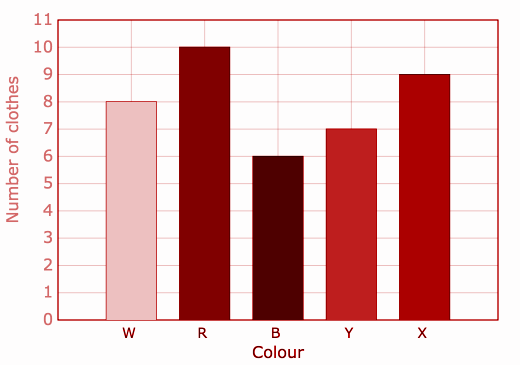Question 3: Payment of the wages for a week in rupees to 30 NREGA workers of Khatwara village are as follows:

830, 835, 890, 810, 815, 816, 814, 845, 898, 890, 819, 860, 832, 817, 855, 845, 804, 808, 812, 816, 885, 835, 815, 812, 878, 840, 868, 890, 806, 828.

Prepare a frequency distribution table having class intervals as 800 – 810, 810 – 820 using tally marks. Draw a histogram using the table.

Solution:

Frequency Distribution Table

 Wages Tally Marks Number of labourers 800 – 810 ||| 3 810 – 820 |||| |||| 10 820 – 830 | 1 830 – 840 |||| 4 840 – 850 ||| 3 850 – 860 | 1 860 – 870 || 2 870 – 880 | 1 880 – 890 | 1 890 – 900 |||| 4 Total 30

Histogram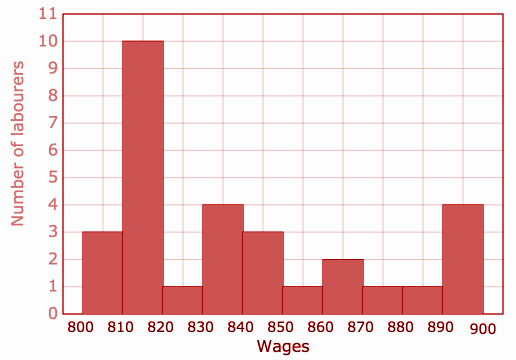Question 4: The number of mobiles sold in 30 days by Ms Raja electronics

222, 228, 238, 215, 225, 219, 217, 230, 218, 237, 214, 210, 235, 222, 220, 214, 212, 220, 237, 212, 238, 218, 210, 216, 217, 234, 233, 237, 219, 239.

Prepare a frequency distribution table having class interval as 210 – 215, 215 – 220 using tally marks. Draw a histogram using a table and answer the following questions.

(i) The number of mobiles sold in which class interval is maximum?

(ii) On how many days 230 or more mobiles were sold?

(iii) On how many days less than 230 or more than 230 mobiles were sold?

Solution:

 Wages Tally Marks Number of labourers 210 – 215 |||| | 6 215 – 220 |||| ||| 8 220 – 225 || 2 225 – 230 ||| 3 230 – 235 |||| 4 235 – 240 |||| || 7 Total 30

Histogram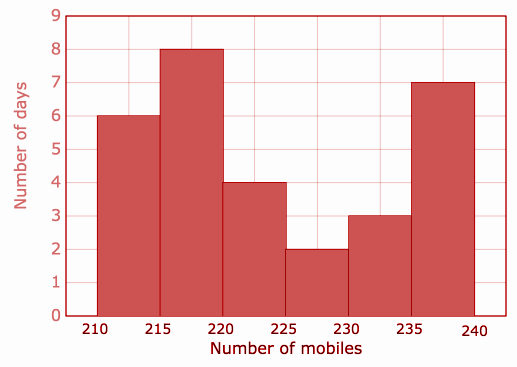(i) The class interval 215 – 220 has the greatest frequency.

(ii) In 3 + 7 = 10 days, 230 or more mobiles are sold.

(iii) In 6 + 8 + 4 + 2 = 20 days, less than 230 mobiles are sold.

Question 5: In holidays the number of study hours every day of students of class 8 is shown in the graph.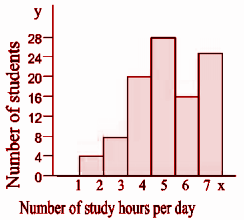(i) How many hours did most of the students study?

(ii) How many students studied for 5 or less than 5 hours?

(iii) How many students studied during vacations?

(iv) Frequency of which class interval is maximum?

Solution:

(i) Maximum students studied for 4 – 5 hours.

(ii) Number of students who studied for 5 or less than 5 hours = 0 + 8 + 20 + 28 = 60.

(iii) Number of students who studied during the holidays = 4 + 8 + 20 + 28 + 16 + 24 = 100.

(iv) The class interval 4 – 5 has the maximum frequency.

### RBSE Maths Chapter 16: Exercise 16.2 Textbook Important Questions and Solutions

Question 1: Number of textbooks of a school is given below. Represent this data through a pie chart.

 Subject Science Maths English Hindi Social Science Total Books 200 120 190 170 40 720

Solution:

 Subject Number of books Number of parts in total Angle formed Science 200 200 / 720 = 5 / 18 [5 / 18] * 360o = 100o Maths 120 120 / 720 = 1 / 6 [1 / 6] * 360o = 60o English 190 190 / 720 = 19 / 72 [19 / 72] * 360o = 95o Hindi 170 170 / 720 = 17 / 72 [17 / 72] * 360o = 85o Social Science 40 40 / 720 = 1 /18 [1 / 18] * 360o = 20o Total 720 720 / 720 = 1 360o

Pie Chart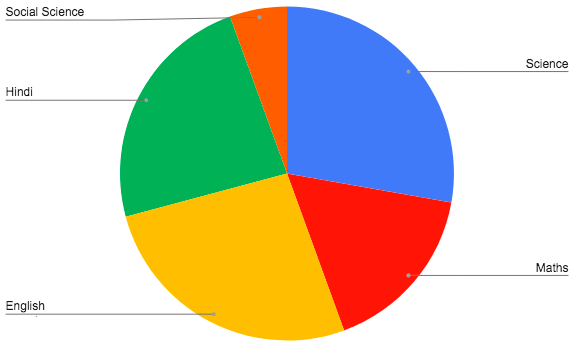Question 2: Description of daily activities of a child is as follows. Represent this data in the form of a pie chart.

 Time Spent School Sleep Play Others Hours 8 6 2 8

Solution:

 Time Spent Hours Number of parts in total Angle formed School 8 8 / 24 = 1 / 3 [1 / 3] * 360o = 120o Sleep 6 6 / 24 = 1 / 4 [1 / 4] * 360o = 90o Play 2 2 / 24 = 1 / 12 [1 / 12] * 360o = 30o Others 8 8 / 24 = 1 / 3 [1 / 3] * 360o = 120o Total 24 1 360o

Pie Chart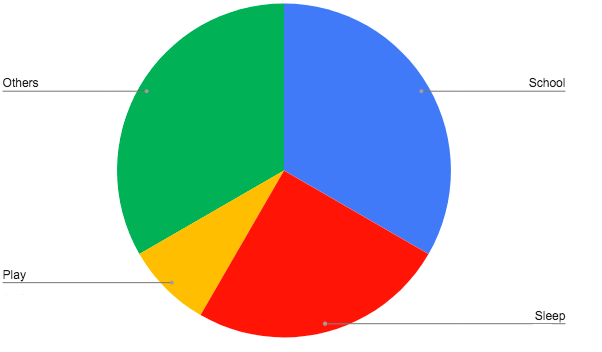Question 3: The below pie chart shows marks obtained by a student in various subjects Hindi, English, Mathematics, Social Science and Science. If the total marks obtained by these students is 900, then answer the following questions.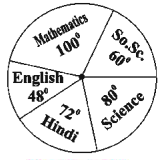(i) In which subject did the student have 250 marks?

(ii) What is the increase in marks obtained by the student between Mathematics and Hindi when compared to each other?

(iii) Check whether total marks obtained in Social Science and Mathematics is more than the total of marks obtained in English and Hindi?

Solution:

(i) Part of whole = 250 / 900

Part of 360o = [250 / 900] * 360o = 100

Hence, the student has obtained 250 marks in Maths.

(ii) Marks obtained in Maths = 250 Marks

Marks obtained in Hindi = [72o / 360o] * 900 = 180

The difference of marks between Maths and Hindi = 250 – 180 = 70

(iii) Marks obtained in Social Science = [60o / 360o] * 900 = 150

Marks obtained in Maths = 250 Marks

Total marks obtained in Social Science and Maths = 150 + 250 = 400

Marks obtained in English = [48o / 360o] * 900 = 120

Marks obtained in Hindi = 180

Total marks obtained in English and Hindi = 120 + 180 = 300

The sum of the marks obtained in Social Science and Maths is greater than the sum of the marks obtained in English and Hindi.

Question 4: Draw a pie chart showing the following information. The table below shows the games liked by students in the class.

 Game Kho-Kho Kabaddi Football Cricket Number of students 5 6 7 18

Solution:

 Game Number of students Number of parts in a whole Angle formed Kho-Kho 5 5 / 36 [5 / 36] * 360o = 50o Kabaddi 6 6 / 36 = 1 / 6 [6 / 36] * 360o = 60o Football 7 7 / 36 [7 / 36] * 360o = 70o Cricket 18 18 / 36 = 1 / 2 [18 / 36] * 360o = 180o Total 36 1 360o

Pie Chart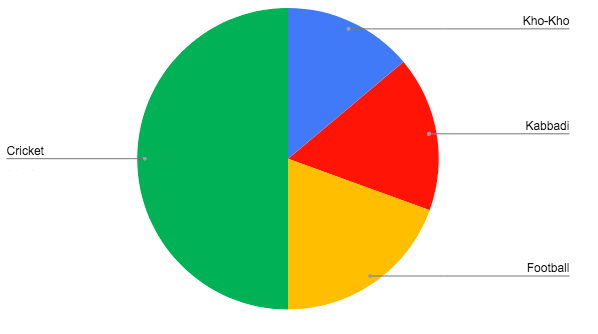Question 5: In accordance with Jan Dhan Yojana of the Indian government, the number of accounts opened by the following banks in one month in Kota city is as follows:

 Name of the bank SBBJ SBI IDBI BOI Number of accounts opened 21000 18000 6000 9000

Draw a pie chart of the above data.

Solution:

 Name of the bank Number of accounts opened Number of parts in a whole Angle formed SBBJ 21000 21000 / 54000 = 7 / 18 [7 / 18] * 360o = 140o SBI 18000 18000 / 54000 = 1 / 3 [1 / 3] * 360o = 120o IDBI 6000 6000 / 54000 = 1 / 9 [1 / 9] * 360o = 40o BOI 9000 9000 / 54000 = 1 / 6 [1 / 6] * 360o = 60o Total 54000 1 360o

Pie Chart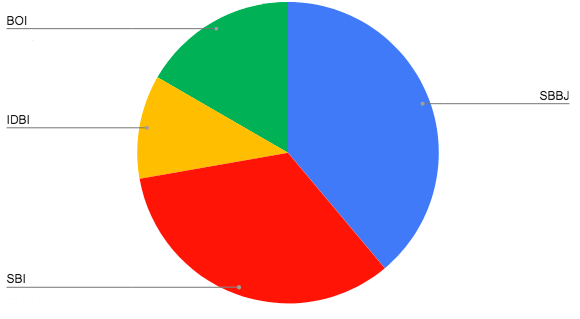Question 6: Various mistakes of automobile drivers are shown in the pie chart.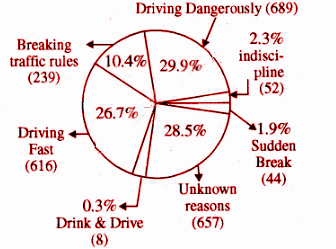With the help of pie chart, answer the following questions-

(i) Percentage of accidents by driving vehicles with fast speed?

(ii) Due to which mistake of an automobile driver, the maximum accidents took place?

(iii) How many accidents occurred due to driving an automobile in a drunken state?

(iv) How many people underwent an accident due to the disobedience of traffic rules?

Solution:

(i) 26.7%

(ii) 29.9% [Dangerous driving]

(iii) 0.3%

(iv) 10.4%

### RBSE Maths Chapter 16: Exercise 16.3 Textbook Important Questions and Solutions

Question 1: What is the probability of getting a head on tossing a coin?

Solution:

The outcomes of tossing a coin are either head or a tail.

Sample space = {H, T}

= [Number of favourable outcomes] / [Total number of outcomes]

= [1 / 2]

Question 2: There are 6 white, 11 red and 7 yellow balls in a bag. What is the probability of getting a white ball from the bag?

Solution:

Total balls = 6 + 11 + 7 = 24

Number of white balls = 6

Probability of getting white ball from the bag

= [Number of favourable outcomes] / [Total number of outcomes]

= 6 / 24

= 1 / 4

Question 3: What is the probability of getting a queen from a pack of well-shuffled cards?

Solution:

Total number of cards = 52

Number of queens = 4

Probability of getting a queen

= [Number of favourable outcomes] / [Total number of outcomes]

= 4 / 52

= 1 / 13

Question 4: Find the probability of each below-mentioned event when a dice is thrown.

(i) A prime number

(ii) No prime number

(iii) Number more than 3

(iv) Number not more than 5

(v) An odd number.

Solution:

The total number of outcomes when a dice is thrown = (1, 2, 3, 4, 5, 6)

(i) Total number of favorable outcomes = 3 (2, 3, 5)

Required probability = 3 / 6 = 1 / 2

(ii) Total number of favorable outcomes = 3 (1, 4, 6)

Required probability = 3 / 6 = 1 / 2

(iii) Number of favorable outcomes = 3 (4, 5, 6)

Required probability = 3 / 6 = 1 / 2

(iv) Number of favorable outcomes = 5 (1, 2, 3, 4, 5)

Required probability = 5 / 6

(v) Number of favorable outcomes = 3 (1, 3, 5)

Required probability = 3 / 6 = 1 / 2

Question 5: Number from 1 to 15 is written on 15 different slips (one number on each slip). They are mixed and kept in a box. A slip is taken out without looking into the box. What is the probability of the following?

(i) Obtaining number 5.

(ii) Obtaining a number with two digits.

(iii) Obtaining a number having one digit.

Solution:

Total no. of slips = 15

Total number of outcomes = 15

(i) Probability of getting a number 5

= [Number of favourable outcomes] / [Total number of outcomes]

= 1 / 15

(ii) Total number of favorable events = 6 (10, 11, 12, 13, 14, 15)

Probability of obtaining a number with two digits

= [Number of favourable outcomes] / [Total number of outcomes]

= 6 / 15 = 2 / 5

(iii) Total number of favorable events = 9 (1, 2, 3, 4, 5, 6, 7, 8, 9)

Probability of obtaining a number having one digit

= [Number of favourable outcomes] / [Total number of outcomes]

= 9 / 15 = 3 / 5

### RBSE Maths Chapter 16: Additional Questions and Solutions

Question 1: Match the following.

 Question Answer Choices 1] Frequency of class 3 – 5 in distribution 4, 8, 3, 6, 7, 2, 3, 5, 9, 4, 6, 5, 5 A] 5 2] Range of class interval 5 – 10 B] 0 3] Probability of getting 8 when a dice is thrown C] ½ 4] Probability of getting a black card from a pack of 52 cards D] 7

Solution:

1] – D]

2] – A]

3] – B]

4] – C]

Question 2: Write any two properties of a histogram

Solution:

• The class intervals are represented by the horizontal line.

• Length of bars indicates the frequency of class intervals.

Question 3: Write the lower and upper limit of class 10 – 20.

Solution:

Lower limit = 10

Upper limit = 20

Question 4: What is the width or size of a class interval?

Solution:

The difference between the upper limit and the lower limit gives the width or size of the class interval.

Question 5: Define the term frequency.

Solution:

The number of times a value that occurs in the data given is called the frequency of the observation.

Question 6: Draw a pie chart for the following data.

 Taste Chocolate Vanilla Other Percentage of the students who like the taste 50 25 25

Solution: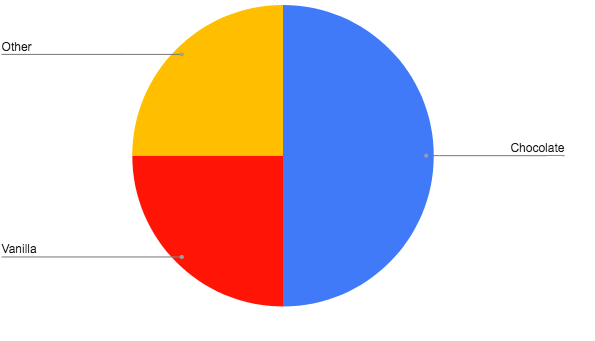Question 7: There are 4 red balls and 2 yellow balls in a bag. What is the probability of drawing a red ball?

Solution:

Total number of outcomes = 4 + 2 = 6

Number of favourable outcomes = 4

Probability of getting a red ball = [Number of favourable outcomes] / [Total number of outcomes]

= 4 / 6

= 2 / 3

Question 8: Find the probability of getting an even number when a dice is thrown.

Solution:

When a dice is thrown, the total outcomes are = 6 (1, 2, 3, 4, 5, 6)

Even number outcomes = 3 (2, 4, 6)

Probability of getting an even number = [Number of favourable outcomes] / [Total number of outcomes]

= 3 / 6

= 1 / 2

Question 9: Draw a proper graph to represent the following data.

 Month Number of watches sold July 1000 August 1500 September 1500 October 2000 November 2500 December 1500

Solution: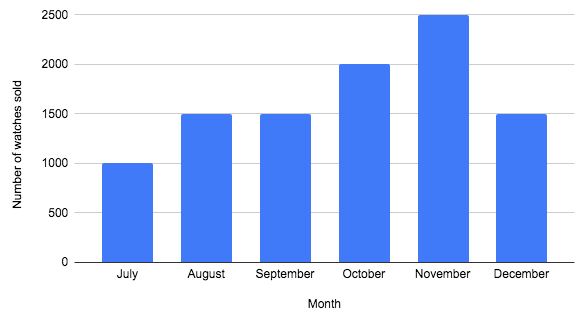Question 10: Observe the histogram given below and answer the following questions.

(i) Which information is being given by histogram?

(ii) In which class interval the number of girls is maximum?

(iii) How many students are having 145 cm or more height?

(iv) If we divide the girls into three categories, then how many girls will be there in each category?

• 150 cm or more – Class A
• Between 140 cm to 150 cm – Class B
• Less than 140 cm – Class C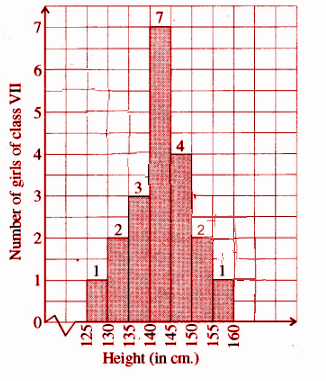Solution:

(i) The histogram represents the height of the students (girls) of class VII.

(ii) In the class interval 140 – 145, the number of girls is maximum.

(iii) The number of students whose height is > or = 145 cm are 4 + 2 + 1 = 7.

(iv) In class A = 2 + 1 = 3 girls

In class B, 7 + 4 = 11 girls

In class C,1 + 2 + 3 = 7 girls

Question 11: From the given bar graph, answer the following questions.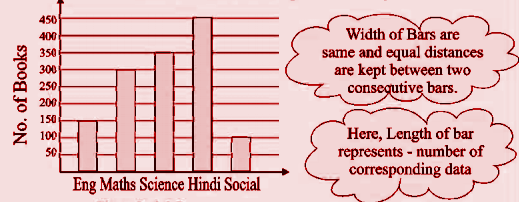(i) Which subject has the maximum number of books and how many?

(ii) Which subject has the least number of books and how many?

(iii) How many books are there in the library?

(iv) What information has been given by this bar graph?

(v) The difference in the number of books of which two subjects is minimum?

Solution:

(i) Hindi = 450

(ii) Social Science = 100

(iii) Total books = 150 + 300 + 350 + 450 + 100 = 1350

(iv) This bar graph represents the number of books of each subject in the library.

(v) Subjects Maths and Science (350 – 300 = 50) and English and Social Science (150 – 100 = 50) have the minimum difference of books.

Question 12: Draw appropriate graphs for the following data.

[i]
 Year 2010 2011 2012 2013 2014 Number of books brought for the library 170 150 190 180 210
[ii]
 Name of village Makadi Akola Rawaliya Khurd Siwadiya Number of men 1800 1700 1800 1500 Number of women 1600 1700 1900 1600

Solution:

[i]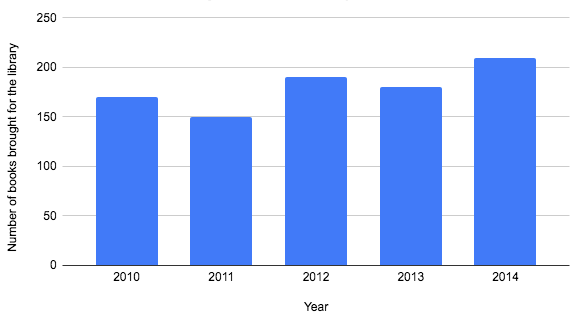[ii]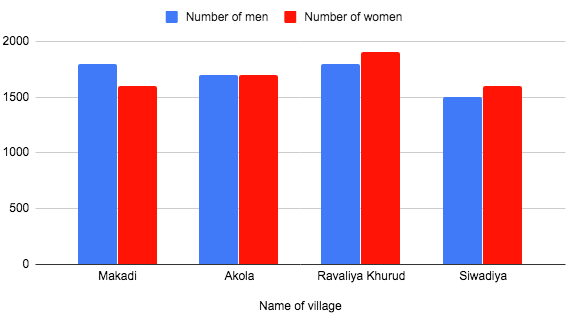Question 13: Study the following frequency distribution and answer the following questions.

 Class interval Frequency 100 – 150 45 150 – 200 25 200 – 250 55 250 – 300 125 300 – 350 140 350 – 400 65 400 – 450 45 Total 500

(i) What is the measure of class interval?

(ii) Which is the upper limit of the class interval 300 – 350?

(iii) Frequency of which group is maximum?

(iv) Frequency of which two groups is the same?

Solution:

(i) Class size 150 – 100 = 50

(ii) 350

(iii) 300-350

(iv) 100 – 150 and 400 – 450